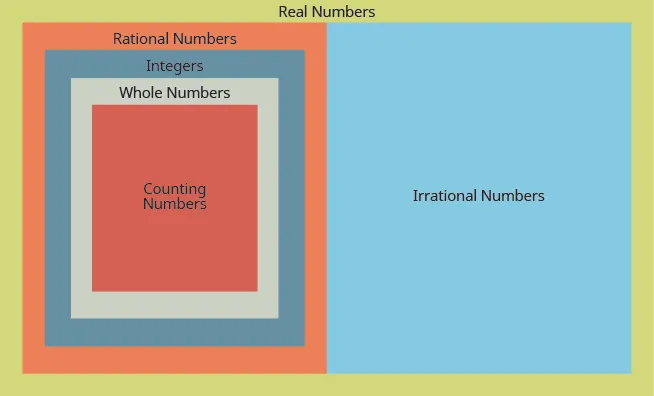Prealgebra 2e

# Key Concepts

Prealgebra 2eKey Concepts

### Key Concepts

#### 7.1Rational and Irrational Numbers

• Real numbers
•#### 7.2Commutative and Associative Properties

• Commutative Properties
• If $a,ba,b$ are real numbers, then $a+b=b+aa+b=b+a$
• Commutative Property of Multiplication:
• If $a,ba,b$ are real numbers, then $a⋅b=b⋅aa⋅b=b⋅a$
• Associative Properties
• If $a,b,ca,b,c$ are real numbers then $(a+b)+c=a+(b+c)(a+b)+c=a+(b+c)$
• Associative Property of Multiplication:
• If $a,b,ca,b,c$ are real numbers then $(a⋅b)⋅c=a⋅(b⋅c)(a⋅b)⋅c=a⋅(b⋅c)$

#### 7.3Distributive Property

• Distributive Property:
• If $a,b,ca,b,c$ are real numbers then
• $a(b+c)=ab+aca(b+c)=ab+ac$
• $(b+c)a=ba+ca(b+c)a=ba+ca$
• $a(b-c)=ab-aca(b-c)=ab-ac$

#### 7.4Properties of Identity, Inverses, and Zero

• Identity Properties
• Identity Property of Addition: For any real number a: $a+0=a0+a=aa+0=a0+a=a$ 0 is the additive identity
• Identity Property of Multiplication: For any real number a: $a⋅1=a1⋅a=aa⋅1=a1⋅a=a$ 1 is the multiplicative identity
• Inverse Properties
• Inverse Property of Addition: For any real number a: $a+(-a)=0-aa+(-a)=0-a$ is the additive inverse of a
• Inverse Property of Multiplication: For any real number a: $(a≠0)a⋅1a=11a(a≠0)a⋅1a=11a$ is the multiplicative inverse of a
• Properties of Zero
• Multiplication by Zero: For any real number a, $a⋅0=00⋅a=0The product of any number and 0 is 0. a⋅0=00⋅a=0The product of any number and 0 is 0.$
• Division of Zero: For any real number a, $0a=0Zero divided by any real number, except itself, is zero. 0a=0Zero divided by any real number, except itself, is zero.$
• Division by Zero: For any real number a, $a0a0$ is undefined and $a÷0a÷0$ is undefined. Division by zero is undefined.
Order a print copy

As an Amazon Associate we earn from qualifying purchases.